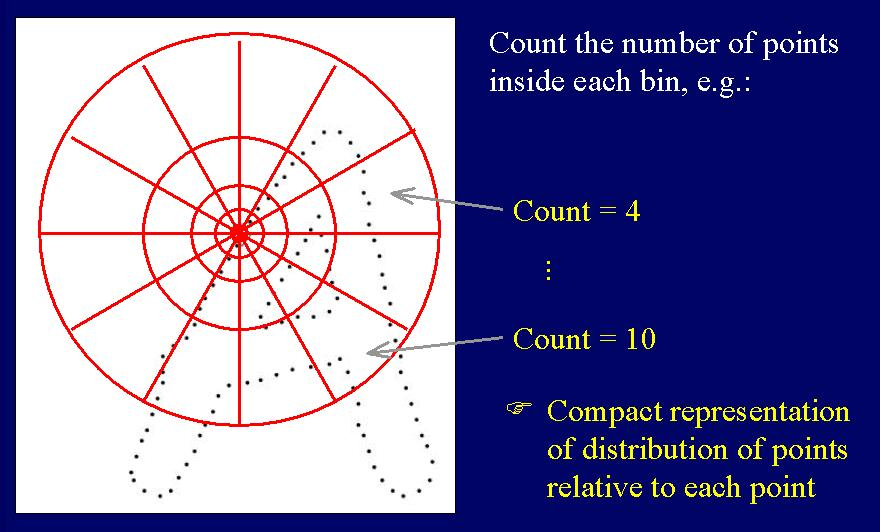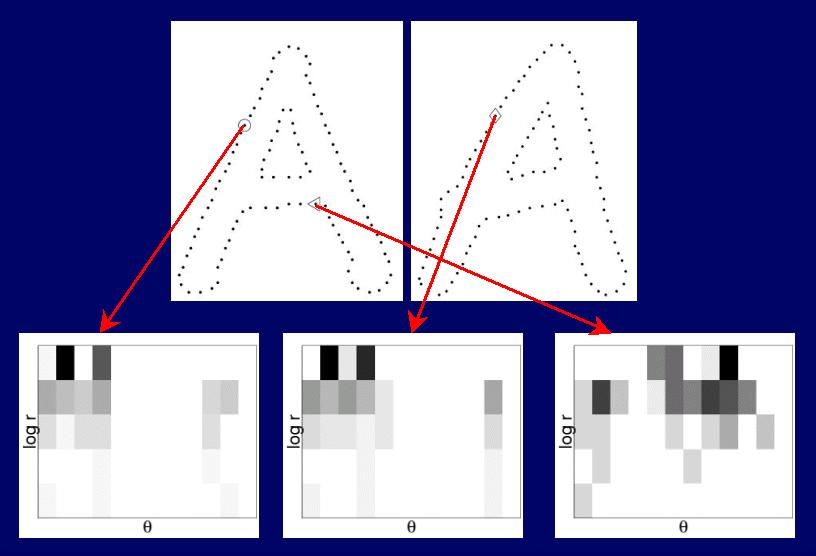# Matching with Shape Contexts

## Serge Belongie, Jitendra Malik and Jan Puzicha

The "shape context" is a new descriptor we have developed for finding correspondences between pointsets. It is described in the following publications:

Matlab demo code for shape context matching with thin plate splines is available here.

Our ICCV 2001 paper contains our record-setting handwritten digit results. This is a problem on which many different algorithms have been compared; see Yann LeCun's algorithm comparison list at AT&T, a representative subset of which is quoted in the following table.

 METHOD TEST ERROR RATE (%) K-nearest-neighbors, Euclidean 5.0 K-nearest-neighbors, Euclidean, deskewed 2.4 40 PCA + quadratic classifier 3.3 1000 RBF + linear classifier 3.6 K-NN, Tangent Distance, 16x16 1.1 Virtual SVM deg 9 poly [distortions] 0.8 Boosted LeNet-4, [distortions] 0.7

The above methods all used a training set of size 60,000. The last two included synthetic distortions of each digit in the training set to produce a training set of size 600,000. Error rates are measured using a test set of size 10,000. Our error rate using shape contexts and a K-nearest-neighbor classifier is 0.63% with a training set of size 20,000.

 METHOD TEST ERROR RATE (%) K-nearest-neighbors, Shape Context [10,000 training] 0.71 K-nearest-neighbors, Shape Context [15,000 training] 0.67 K-nearest-neighbors, Shape Context [20,000 training] 0.63

## Background

The basic idea behind shape contexts is illustrated in the following diagrams. Given a set of points from an image (e.g. extracted from a set of detected edge elements), the shape context captures the relative distribution of points in the plane relative to each point on the shape. Specifically, we compute a histogram using log-polar coordinates as shown here:Thus we get descriptors that are similar for homologous (corresponding) points and dissimilar for non-homologous points, as illustrated here, where the bin counts in the histogram are indicated by the grayshade (black=large, white=small):We then use the shape contexts as attributes for a weighted bipartite matching problem.

More to come...

Last updated October 23, 2001, by Greg Mori